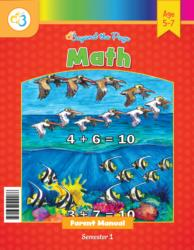# Common Core Alignment

CCSS.Math.Content.1.MD.4 - Organize, represent, and interpret data with up to three categories; ask and answer questions about the total number of data points, how many in each category, and how many more or less are in one category than in another.

## 5: MathUnit 4: Data and Graphing
Lesson 2: Tally Sticks and Tally Marks
Lesson 3: Pictographs
Lesson 4: Bar Graphs
Lesson 5: More Graphing Practice